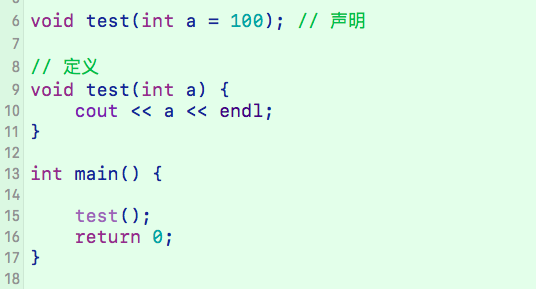C++ 零碎知识点集合

C/C++ 新旧风格

1. C 旧风格
.h 结尾，如 math.h ，由于 C++ 兼容 C ，所以 C/C++ 均可使用。
2. C++ 旧风格
.h 结尾，如 iostream.h ，此时无 名字空间 等特性。
3. C 新风格
前缀加上 c ，无 .h 的扩展名，如 math.h 改为了 cmath ，增加了新特性，可以使用不是 C 的特性，如 namespace std
4. C++ 新风格
iostream ，增加了使用 名字空间(namespace std) 的特性。

命名空间

namespace 即“命名空间”，也称“名称空间” 、”名字空间”。C++ 使用命名空间的原因主要是，人类可用的单词数太少，并且不同的人写的程序不可能所有的变量都没有重名现象，为了解决变量重名，于是引入命名空间来区分，将变量限制在不同的域内。

https://blog.csdn.net/qq_40873884/article/details/79576045

缺省参数（默认参数）

C++ 引入函数缺省参数，即默认参数，极大地提高了使用函数的灵活性，

#include <iostream>
using namespace std;

int test(int x = 3, int y = 5) {
return x + y;
}

int main(int argc, const char * argv[]) {
int a = 1, b = 2;
cout << "传两个参数：" << test(a, b) << endl;
cout << "传一个参数：" << test(a) << endl;
cout << "不传参数：" << test() << endl;
return 0;
}

1. 全缺省参数
2. 半缺省参数

int test(int x = 3, int y = 5);

int test(int x, int y, int z = 1);函数重载

https://blog.csdn.net/qq_40873884/article/details/79827796

引用变量

https://blog.csdn.net/qq_40873884/article/details/79632314